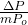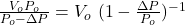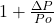## Suppose you have 10 helium-filled party balloons tied to a digital camera, so you can take photos of the core of a storm. With 10 balloons t

Question

Suppose you have 10 helium-filled party balloons tied to a digital camera, so you can take photos of the core of a storm. With 10 balloons the camera will slowly but surely rise. As a storm approaches the atmospheric pressure drops. Assuming the air temperature and density are the same, the volume of each balloon will increase. When you let go, the speed of ascent will be

in progress 0
2 months 2021-07-31T22:53:37+00:00 1 Answers 2 views 0

a = 10 ρ_air gExplanation:

Let’s solve this problem in parts, with the initial data the camera rises slowly, so we can assume that at constant speed, we apply the equilibrium condition

B – W = 0

The thrust is given by Archimedes’ law

B = rho_aire g V_body

The volume of the body can be found from the ideal gas ratio

P V = n R T

let’s use the subscript “o” for the initial concisions

V₀ = (nR) T₀/P₀               1

we substitute

10 ρ_air g (nR) T₀ /P₀ = W

when the storm approaches the pressure decreases, P

If we use the ideal gas equation

V = (nR) T₀ / P

we combine this equation with equation 1

V = V₀P₀ / P

if we write the pressure

P = P₀ -ΔP

we substitute

V =we expand serie  and eliminate higher order terms

V = V₀ ()

with this expression we can write the thrust

B = B₀ + ΔB

Newton’s second law for the new conditions is

B – W = m a

(B₀ + ΔB) – W = ma

ΔB = m a

a = ΔB / m

a = 10 ρ_air gThis is the initial acceleration of the camera